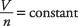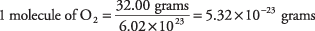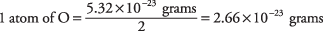The volume of a gas is determined not only by the pressure and volume but also by the quantity of gas. When the quantity is given in moles, the mathematical relation iswhere n represents the number of moles of the gas. This relationship is known as Avogadro's law because, in 1811, Amedeo Avogadro of Italy proposed that equal volumes of all gases contain the same number of molecules. His theory explained why the volumes of gases in reactions are in ratios of small integers, as in the combustion of hydrogen:You should recall from the review of the mole unit that the reaction coefficients (2, 1, and 2 in the preceding example) can be interpreted as molecules, or as moles, or as volumes. This is because Avogadro's law holds for all gases. The number of molecules in 1 mole of a gas is known to be 6.02 × 10 23, a value called Avogadro's number. As an example of the use of this important number, calculate the mass in grams of a single oxygen atom:

1 mole O 2 = 2(16.00) = 32.00 grams• How many hydrogen atoms are there in 10 grams of methane, CH 4?